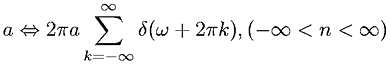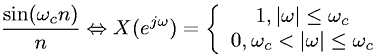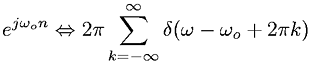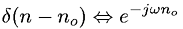Signal Processing > Fourier Transform PairsShowing results 1 to 9 of 9, on page 1 of 1
 DESCRIPTION EQUATION Discrete-Time Fourier transform of a boxcar sequenceDiscrete-Time Fourier transform of a constantDiscrete-Time Fourier transform of a sinc sequenceDiscrete-Time Fourier transform of complex exponentialDiscrete-Time Fourier transform of deltaDiscrete-Time Fourier transform of shifted deltaDiscrete-Time Fourier transform of unit step functionDiscrete-Time Fourier transform of unit step function and exponentialDiscrete-Time Fourier transform of unit step function and exponential